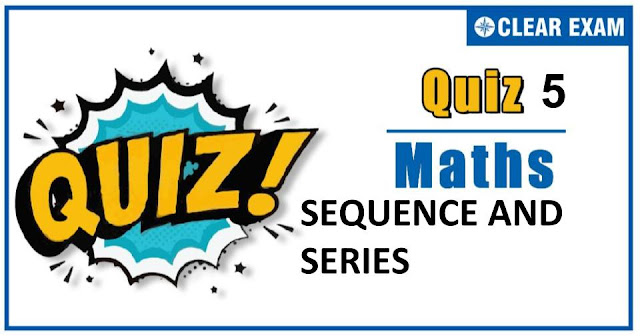## [LATEST]\$type=sticky\$show=home\$rm=0\$va=0\$count=4\$va=0

As per analysis for previous years, it has been observed that students preparing for JEE MAINS find Mathematics out of all the sections to be complex to handle and the majority of them are not able to comprehend the reason behind it. This problem arises especially because these aspirants appearing for the examination are more inclined to have a keen interest in Mathematics due to their ENGINEERING background.

Furthermore, sections such as Mathematics are dominantly based on theories, laws, numerical in comparison to a section of Engineering which is more of fact-based, Physics, and includes substantial explanations. By using the table given below, you easily and directly access to the topics and respective links of MCQs. Moreover, to make learning smooth and efficient, all the questions come with their supportive solutions to make utilization of time even more productive. Students will be covered for all their studies as the topics are available from basics to even the most advanced.

Q1. If the arithmetic mean of a and b is (a^n+b^n)/(a^(n-1)+b^(n-1) ), then the value of n is
•  -1
•  0
•  1
•  None of these
Solution
Since, (a+b)/2=(a^n+b^n)/(a^(n-1)+b^(n-1) ) ⇒(a+b)(a^(n-1)+b^(n-1) )=2(a^n+b^n) ⇒(ab^n)/b+(ba^n)/a=a^n+b^n ⇒ a^n ((a-b)/a)=-b^n ((b-a)/b) ⇒ a^n/b^n =a/b ⇒n=1

Q2.The sum of the first and third term of an arithmetic series is 12 and the product of first and second term is 24, then first term is
•  1
•  8
•  4
•  6
Solution
Let the first three terms of an AP are a-d,a and a+d Since, (a-d)+(a+d)=12 ⇒ a=6 and a(a-d)=24 ⇒ 6-d=4 ⇒ d=2 ∴ First term is a-d=4

Q3.  The sum of the series 1+(1+a)/(2 !)+(1+a+a^2)/(3 !)+(1+a+a^2+a^3)/(4 !)+⋯, is
•   (e^a-e)/(a-1)
•   (e^a-e)/(a+1)
•  (e^2a+1)/(a-1)
•   (e^a+e)/(a-1)
Solution
We have, 1+(1+a)/(2 !)+(1+a+a^2)/(3 !)+(1+a+a^2+a^3)/(4 !)+⋯∞ =∑_(n=1)^∞▒(1+a+a^2+⋯+a^(n-1))/(n !) =∑_(n=1)^∞▒1/(n !) ((1-a^n)/(1-a)) =1/((1-a)) {∑_(n=1)^∞▒1/(n !)-∑_(n=1)^∞▒a^n/(n !)} =1/(1-a) {(e-1)-(e^a-1)}=(e-e^a)/(1-a)=(e^a-e)/(a-1)

Q4. If (a+b)/(1-ab),b,(b+c)/(1-bc) are in A.P., then a,1/b,c are in
•  A.P
•  G.P
•  H.P
•  None of these
Solution
We have, (a+b)/(1-ab),b,(b+c)/(1-bc) are in A.P. ⇒b-(a+b)/(1-ab)=(b+c)/(1-bc)-b ⇒-a(b^2+1)/(1-ab)=(c(b^2+1))/(1-bc) ⇒-((1-ab)/a)=(1-bc)/c ⇒-1/a+b=1/c-b ⇒2 b=1/a+1/c⇒a,1/b,c are in HP

Q5.2^sin⁡〖θ 〗 +2^cos⁡θ is greater than
•  1/2
•  √2
•  2^(1/√2)
•  2^((1-1/√2) )
Solution

Q6. If a(1/b+1/c),b(1/c+1/a),c(1/a+1/b) are in A.P., then
•  a,b,c are in A.P.
•  1/a,1/b,1/c are in A.P.
•  a,b,c are in H.P.
•  1/a,1/b,1/c are in G.P
Solution

Q7.The coefficient of n^(-r) in the expansion of log_10⁡〖(n/(n-1)),〗 is
•  1/(r log_e⁡10 )
•   -1/(r log_e⁡10 )
•  -1/(r !log_e⁡10 )
•  log_e⁡(1-1/n)/log_e^0
Solution
We have, log_10⁡〖(n/(n-1))=log_e⁡〖(n/(n-1))∙log_10⁡e 〗 〗 ⇒log_10⁡〖(n/(n-1))=-log_e⁡((n-1)/n) ∙log_10⁡e 〗 ⇒log_10⁡〖(n/(n-1))〗=-log_10⁡e∙log_e⁡(1-1/n) ⇒log_10⁡〖(n/(n-1))=log_10⁡〖e {∑_(r=1)^∞▒〖1/r (1/n)^r 〗}=〗 〗 ∑_(r=1)^∞▒〖{1/r log_10⁡e } n^(-r) 〗 ∴ Coefficient of n^(-r)=1/r log_10⁡〖e=1/(r log_e⁡10 )〗

Q8.1+log_2⁡〖2+(log_e⁡2 )^2/(2 !)+(log_e⁡2 )^3/(3 !)+⋯〗 is equal to
•  2
•  1/2
•  log_e⁡3
•  None of these
Solution
We have, 1+log_e⁡2+(log_e⁡2 )^2/2!+(log_e⁡2 )^3/3!+⋯ =e^log_e⁡2 =2

Q9.If the sum of the first n natural numbers is 1/5 times the sum of their squares, then the value of n is
•  5
•  6
•  7
•  8
Solution
We have, ∑▒〖n=(1/5) ∑▒n^2 〗 ⇒n ((n+1))/2=1/5 {n(n+1)(2 n+1)/6} ⇒2 n+1=15 ⇒n=7

Q10. If the m^th,n^th and p^th terms of an A.P. and G.P. be equal and be respectively x,y,z, then
•  x^y y^z z^x=x^z y^x z^y
•  (x-y)^x (y-z)^y=(z-x)^z
•  (x-y)^z (y-z)^x=(z-x)^y
•   None of these
Solution#### Written by: AUTHORNAME

AUTHORDESCRIPTION## Want to know more

Please fill in the details below:

## Latest NEET Articles\$type=three\$c=3\$author=hide\$comment=hide\$rm=hide\$date=hide\$snippet=hide

Name

ltr
item
BEST NEET COACHING CENTER | BEST IIT JEE COACHING INSTITUTE | BEST NEET, IIT JEE COACHING INSTITUTE: SEQUENCE AND SERIES QUIZ-5
SEQUENCE AND SERIES QUIZ-5
https://1.bp.blogspot.com/-fdNJXP-omDE/X7EI_T5dqzI/AAAAAAAAN04/JDey0bpGQgomQvzuBue95ILIhege5-9_gCLcBGAsYHQ/w640-h336/Quiz%2BImage%2B20%2B%25286%2529.jpg
https://1.bp.blogspot.com/-fdNJXP-omDE/X7EI_T5dqzI/AAAAAAAAN04/JDey0bpGQgomQvzuBue95ILIhege5-9_gCLcBGAsYHQ/s72-w640-c-h336/Quiz%2BImage%2B20%2B%25286%2529.jpg
BEST NEET COACHING CENTER | BEST IIT JEE COACHING INSTITUTE | BEST NEET, IIT JEE COACHING INSTITUTE
https://www.cleariitmedical.com/2020/11/sequence%20and%20series%20quiz%205.html
https://www.cleariitmedical.com/
https://www.cleariitmedical.com/
https://www.cleariitmedical.com/2020/11/sequence%20and%20series%20quiz%205.html
true
7783647550433378923
UTF-8